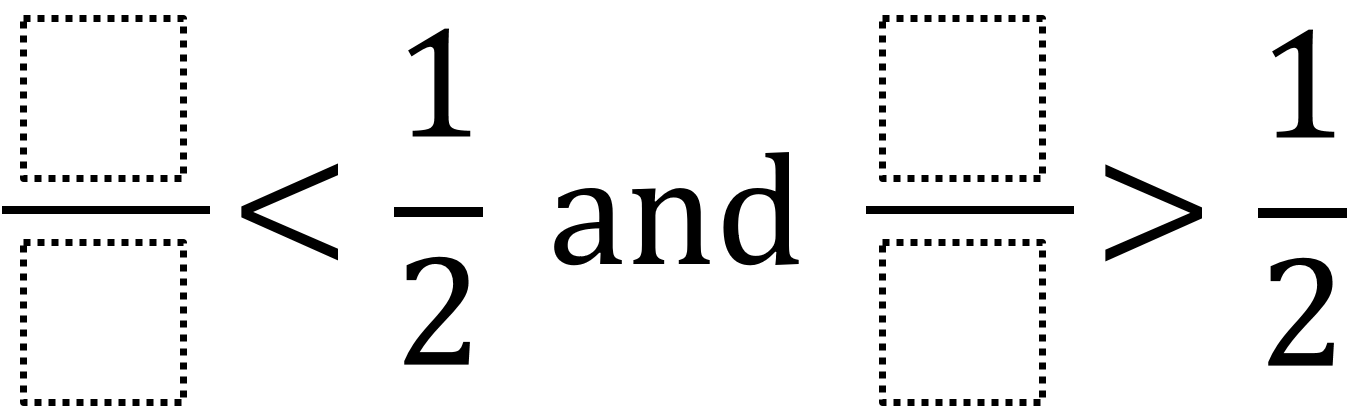# Comparing Fractions

Directions: Use the digits 1 to 9, at most one time each, to fill in the boxes to create two different fractions: one that is less than one half and one that is more than one half.### Hint

How can we tell when a fraction is less than one half?
How can we find a fraction that is less than one half but has a numerator larger than 1?
How can we tell when a fraction is more than one half?

There are many possible answers including

1/4 and 8/9

Other answers you may not expect would be improper fractions that are greater than 1/2 such as 5/2.

Source: Robert Kaplinsky

## Comparing Fractions

Directions: Using the numbers 1-9, each one time only, fill in the blanks to make …

1.John Birnstengel

1/5 and 7/8

2.1/8 and 3/4

3.4.It could be 1/41/2.

•Sorry, there was a glitch. I meant to say that 1/4 is less than 1/2 and 3/4 is greater than 1/2.